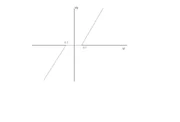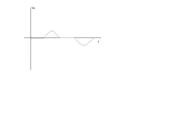133 Pages

## CourseEdit

This page is for the course ELEC3106 - Electronics and is based on the lectures of Torsten Lehman senior lecturer at the University of New South Wales.

## OverviewEdit

Non linearity is a situation where a real life analogue amplifier is unable to produce an output voltage which linearily follows the input voltage. This is of concern when designing an amplifier as a non linear amplifier has the following traits:

• Input signal is distorted, this means the output signal can't reflect the input signal making the amplifier essentially useless.
• The designer is unable to accurately predict the output of a non linear circuit.
• The circuit is unable to measure or receive signals with accuracy

## PrerequisitesEdit

The reader of this page is assumed to have a basic mathematical knowledge (concerning linearity), and a basic knowledge of analogue circuitry, such as that gained from the course ELEC2133 - Analogue Electronics.

## Non LinearityEdit

### Causes and EffectsEdit

In this section the various causes of non linearity are discussed as well as their effect on the output signal of the amplifier. Note that unless shown otherwise in the figures the input voltage is a pure sine wave.

Clipping

Clipping is where the output voltage is so high that it reaches the rails of the circuit and is unable to exceed these, and as such the signal is distorted. This is shown pictorially in figure 1. Clipping is caused by the amplifier having a gain which is too large, thus causing Vout beFigure 2: illustration of how bias currents create non linearity outside the range, or having an input signal which is too large for the amplifier, again causing Vout to be too large.

Crossover Distortion

Is caused by the bias voltages in the transistors that make up the amplifier. This causes a gap where the ouput voltage is zero and as such is unable to follow the input voltage, this is shown in figure 2. The effect of this is a non linear distortion of the input which is even worse if the signal is small, as shown in figure 3.Figure 3: Effect of crossover distortion on a small signal

Soft Saturation

Soft saturation is an effect where the output voltage is slowly saturated in either a positive or negative sense. This effect produces a non linear ouFigure 4: Soft saturation and its effect on a sine wave tput as shown in figure 4.

### Quantification of non linear circuitsEdit

To quanitify non linear circuits we examine the ouput voltage in the frequency domain. This is because it is very hard to accurately put a number on non linearity in the time domain. The main method of quanitfying non linear circuits is to claculate the total harmonic distortion (THD). This is done by plotting the frequency response of the output and measuring the peaks as V1, V2, ..., VN, where V1 is the fundamental frequency (the expected frequency). We then use the following equation:

$\mathrm{THD} = \sqrt{\frac{V_2^2 + V_3^2 + \ldots + V_N^2}{V_1^2}} = \frac{1}{V_1}\left(\sum_{i=2}^N{V_i^2}\right)^{1/2}$

Usually the THD is expressed as a percentage. Typical values are around 1%.

### Fixing Non LinearityEdit

One very good method to make a circuit linear is to incorporate a high gain negative feedback into the circuit. The effect of this is that Vo is brought closer in line to Vin by the feedback mechanism, in much the same way as feedback eliminates noise. See figure 5 for an example.

## Further ReadingEdit

It is recommended that the reader visits https://subjects.ee.unsw.edu.au/elec3106/ and reads the lecturers scribbles as an alternative source.

## See AlsoEdit

The page on electrical noise coming to an elsoc.wikia page near you!

Community content is available under CC-BY-SA unless otherwise noted.